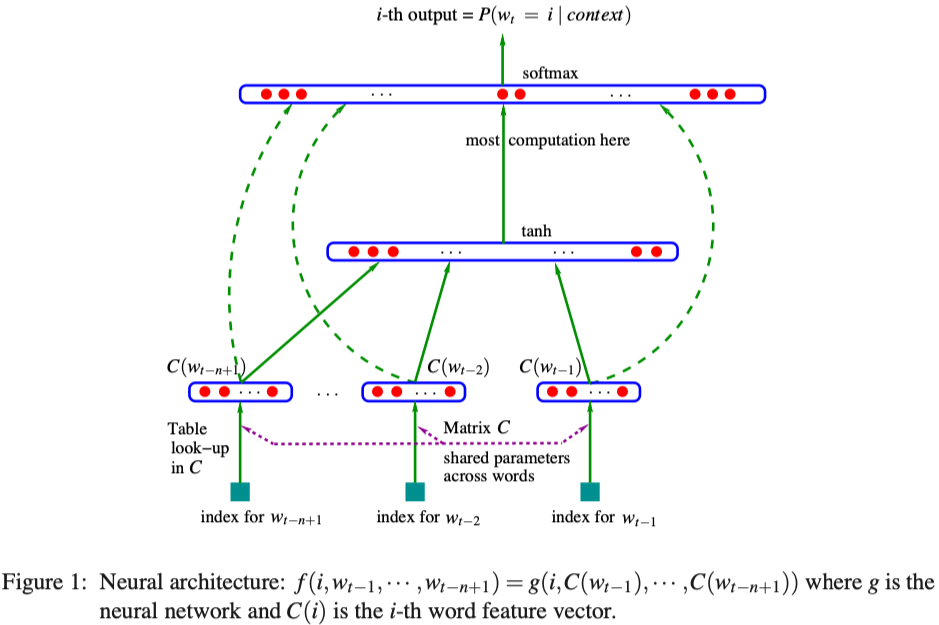# Understanding Neural Probabilistic Language Model

$\newcommand{\argmin}{\mathop{\mathrm{argmin}}\limits}$ $\newcommand{\argmax}{\mathop{\mathrm{argmax}}\limits}$

Neural Probabilistic Language Model (NPLM for short; Bengio et al., 2003) was a turning point when it comes to word embedding. Based on the n-gram language model and as an end-to-end model it proved that a neural network trained on predicting the following word given n-gram can be useful in embedding lexical context into vectors.

## Background

To generalize the embedding result to not only the already-seen sentences, but to sentences containing words that are not used in training, distributing the probability mass of a point (sentence) to its neighbors (similar sentences), so that the distribution becomes somewhat continuous, is required. Previous to NPLM, this has been achieved by (1) approximating n-grams with m-grams with $m<n$ (which is called back-off technique) (2) adding constant $k$ to each n-gram frequency (add-$k$ smoothing) or (3) interpolation.

However, these techniques have not used the context farther than $n$ words. In addition, previous models does not embeds semantic and/or grammatical similarity between words. Bengio et al. (2003) proposed a neural net based method that account the points as follows.

1. associate with each word in the vocabulary a distributed word feature vector (a real-valued vector in $\mathbb{R}^m$),
2. express the joint probability function of word sequences in terms of the feature vectors of these words in the sequence, and
3. learn simultaneously the word feature vectors and the parameters of that probability function.

Here, word feature vector refers to word embedding and the probability function refers to the neural net they will about to implement in the paper. They improved the performance by not using the vanilla n-grams but by embedding semantic/grammatical usage of each word into real-valued vectors.

The architecture of the neural net is as follows (Bengio et al., 2003).It is in essence a simple feed-forward network using only fully-connected layers.

## Implementation

### with PyTorch

Each sample is as follows:

{
"text": ["<(t-3)th word>", "<(t-2)th word>", "<(t-1)th word>"],
"label": "<t-th word>"
}


The model

$y = b + Wx + U \tanh(d + Hx)$

where $W, b$ are parameters of the first affine layer and $H, d$ are of the hidden affine layer, $U$ is of the another affine layer, can be implemented as follows:

import torch
import torch.nn as nn
import torch.nn.functional as F

class NPLM(nn.Module):

def __init__(self, vocab_size, n_gram, embedding_dim, hidden_dim):
super(NPLM, self).__init__()
self.vocab_size = vocab_size
self.n_gram = n_gram
self.embedding_dim = embedding_dim
self.hidden_dim = hidden_dim

# embedding
self.embedding = nn.Embedding(vocab_size, embedding_dim)
# affine layers for tanh
self.linear1 = nn.Linear(n_gram * embedding_dim, hidden_dim)
self.linear2 = nn.Linear(hidden_dim, vocab_size, bias=False)
# affine layer for residual connection
self.linear3 = nn.Linear(n_gram * embedding_dim, vocab_size)

def forward(self, x):
x = self.embedding(x)
#print(x.shape)

x = x.view(-1, self.embedding_dim * self.n_gram)
#print(x.shape)

x1 = torch.tanh(self.linear1(x))
x1 = self.linear2(x1)

x2 = self.linear3(x)

x = x1 + x2
#print(x.shape)

return x


Define loss function and optimizer.

# create an instance of NPLM
model = NPLM(VOCAB_SIZE, N_GRAM, EMBEDDING_DIM, HIDDEN_DIM)

# use cross entropy loss, SGD optimizer with momentum
criterion = nn.CrossEntropyLoss()
optimizer = optim.SGD(model.parameters(), lr=0.01, momentum=0.9)

# train on CUDA GPU if available
device = torch.device("cuda" if torch.cuda.is_available() else "cpu")

model = model.to(device)
criterion = criterion.to(device)


Then train the model.

def accuracy(pred, target):
"""
calculate accuracy as a ratio of n_correct:total.
"""
pred = torch.argmax(torch.softmax(pred, dim=1), dim=1)
correct = (pred == target).float()

return correct.sum() / len(correct)

def train(model, iterator, criterion, optimizer):
"""
iterator: torchtext.data.BucketIterator object.
"""
loss_epoch = 0.
acc_epoch = 0.
for batch in trainiter:
model.zero_grad()

out = model(batch.text)
out = out.squeeze(0)

target = batch.label.squeeze(0)

loss = criterion(out, target)
loss.backward()
optimizer.step()

loss_epoch += loss.item()
acc_epoch += accuracy(out, target).item()
return loss_epoch, acc_epoch

N_EPOCH = 200

losses = []
accs = []
for i in range(1, N_EPOCH+1):
loss_epoch, acc_epoch = train(model, trainiter, criterion, optimizer)
losses.append(loss_epoch)
accs.append(acc_epoch)

if i % 5 == 0:
print(f"epoch: {i:03}, loss: {loss_epoch/len(trainiter): .3f}, acc: {acc_epoch/len(trainiter): .4f}")

torch.save(model.state_dict(), "./NPLM_SGD_lr0.01_momentum0.9_epoch200.pth")


### with TensorFlow (v2.4)

Similarly, it is not difficult to implement NPLM with tf.keras.

import tensorflow as tf

class NPLM(tf.keras.Model):

def __init__(self, vocab_size, context_size, embedding_dim, hidden_dim):
super(NPLM, self).__init__()
self.embedding = tf.keras.layers.Embedding(vocab_size, embedding_dim)
# affine layers for tanh
self.flatten = tf.keras.layers.Flatten()
self.linear1 = tf.keras.layers.Dense(hidden_dim, activation="tanh")
self.linear2 = tf.keras.layers.Dense(vocab_size, use_bias=False)
# affine layer for residual connection
self.linear3 = tf.keras.layers.Dense(vocab_size)

def call(self, x):
x = self.embedding(x)
x = self.flatten(x)

x1 = self.linear1(x)
x1 = self.linear2(x1)

x2 = self.linear3(x)

x = x1 + x2
return x


Full result of each implementation can be seen here (Gist).

References

• Bengio et al. 2003. A neural probabilistic language model. The journal of machine learning research, 3, 1137-1155.
• Lee. 2019. 한국어 임베딩 (Embedding Korean). 에이콘 출판사.